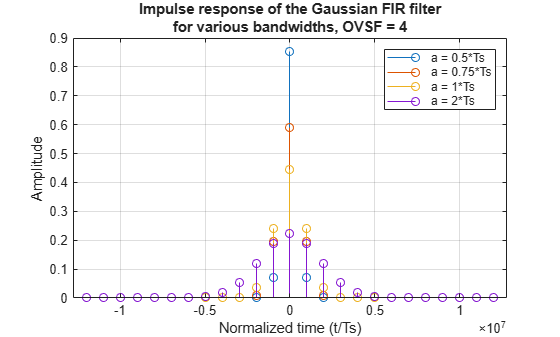# FIR Gaussian Pulse-shaping Filter Design

This example shows how to design a Gaussian pulse-shaping FIR filter and the parameters influencing this design. The FIR Gaussian pulse-shaping filter design is done by truncating a sampled version of the continuous-time impulse response of the Gaussian filter which is given by:

`$h\left(t\right)=\frac{\sqrt{\pi }}{a}{e}^{-\frac{{\pi }^{2}{t}^{2}}{{a}^{2}}}$`

The parameter 'a' is related to 3-dB bandwidth-symbol time product (B*Ts) of the Gaussian filter as given by:

`$a=\frac{1}{B{T}_{s}}\sqrt{\frac{\mathrm{log}2}{2}}$`

There are two approximation errors in this design: a truncation error and a sampling error. The truncation error is due to a finite-time (FIR) approximation of the theoretically infinite impulse response of the ideal Gaussian filter. The sampling error (aliasing) is due to the fact that a Gaussian frequency response is not really band-limited in a strict sense (i.e. the energy of the Gaussian signal beyond a certain frequency is not exactly zero). This can be noted from the transfer function of the continuous-time Gaussian filter, which is given as below:

`$H\left(f\right)={e}^{-{a}^{2}{f}^{2}}$`

As f increases, the frequency response tends to zero, but never is exactly zero, which means that it cannot be sampled without some aliasing occurring.

### Continuous-time Gaussian Filter

To design a continuous-time Gaussian filter, let us define the symbol time (Ts) to be 1 micro-second and the number of symbols between the start of the impulse response and its end (filter span) to be 6. From the equations above, we can see that the impulse response and the frequency response of the Gaussian filter depend on the parameter 'a' which is related to the 3 dB bandwidth-symbol time product. To study the effect of this parameter on the Gaussian FIR filter design, we will define various values of 'a' in terms of Ts and compute the corresponding bandwidths. Then, we will plot the impulse response for each 'a' and the magnitude response for each bandwidth.

```Ts = 1e-6; % Symbol time (sec) span = 6; % Filter span in symbols a = Ts*[.5, .75, 1, 2]; B = sqrt(log(2)/2)./(a); t = linspace(-span*Ts/2,span*Ts/2,1000)'; hg = zeros(length(t),length(a)); for k = 1:length(a) hg(:,k) = sqrt(pi)/a(k)*exp(-(pi*t/a(k)).^2); end plot(t/Ts,hg) title({'Impulse response of a continuous-time Gaussian filter';... 'for various bandwidths'}); xlabel('Normalized time (t/Ts)') ylabel('Amplitude') legend(sprintf('a = %g*Ts',a(1)/Ts),sprintf('a = %g*Ts',a(2)/Ts),... sprintf('a = %g*Ts',a(3)/Ts),sprintf('a = %g*Ts',a(4)/Ts)) grid on;```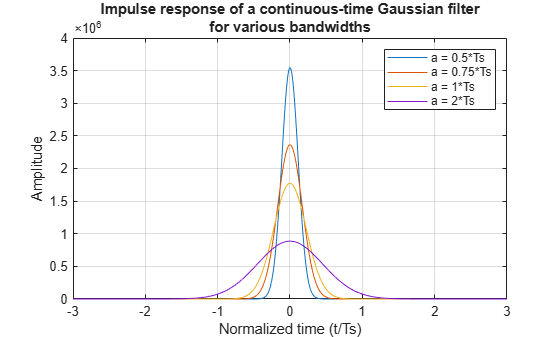Note that the impulse responses are normalized to the symbol time.

### Frequency Response for Continuous-time Gaussian Filter

We will compute and plot the frequency response for continuous-time Gaussian filters with different bandwidths. In the graph below, the 3-dB cutoff is indicated by the red circles ('o') on the magnitude response curve. Note that 3-dB bandwidth is between DC and B.

```f = linspace(0,32e6,10000)'; Hideal = zeros(length(f),length(a)); for k = 1:length(a) Hideal(:,k) = exp(-a(k)^2*f.^2); end plot(f,20*log10(Hideal)) titleStr = {'Ideal magnitude response for a continuous-time ';... 'Gaussian filter for various bandwidths'}; title(titleStr); legend(sprintf('B = %g',B(1)),sprintf('B = %g',B(2)),... sprintf('B = %g',B(3)),sprintf('B = %g',B(4))) hold on for k = 1:length(a) plot(B,20*log10(exp(-a.^2.*B.^2)),'ro','HandleVisibility','off') end axis([0 5*max(B) -50 5]) xlabel('Frequency (Hz)') ylabel('Magnitude (dB)') grid on;```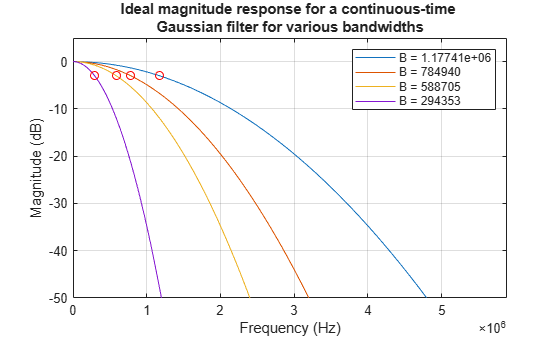### FIR approximation of the Gaussian Filter

We will design the FIR Gaussian filter using the gaussdesign function. The inputs to this function are the 3-dB bandwidth-symbol time product, the number of symbol periods between the start and end of the filter impulse response, i.e. filter span in symbols, and the oversampling factor (i.e. the number of samples per symbol).

The oversampling factor (OVSF) determines the sampling frequency and the filter length and hence, plays a significant role in the Gaussian FIR filter design. The approximation errors in the design can be reduced with an appropriate choice of oversampling factor. We illustrate this by comparing the Gaussian FIR filters designed with two different oversampling factors.

First, we will consider an oversampling factor of 16 to design the discrete Gaussian filter.

```ovsf = 16; % Oversampling factor (samples/symbol) h = zeros(97,4); iz = zeros(97,4); for k = 1:length(a) BT = B(k)*Ts; h(:,k) = gaussdesign(BT,span,ovsf); [iz(:,k),t] = impz(h(:,k)); end figure('Color','white') t = (t-t(end)/2)/Ts; stem(t,iz) title({'Impulse response of the Gaussian FIR filter for ';... 'various bandwidths, OVSF = 16'}); xlabel('Normalized time (t/Ts)') ylabel('Amplitude') legend(sprintf('a = %g*Ts',a(1)/Ts),sprintf('a = %g*Ts',a(2)/Ts),... sprintf('a = %g*Ts',a(3)/Ts),sprintf('a = %g*Ts',a(4)/Ts)) grid on;```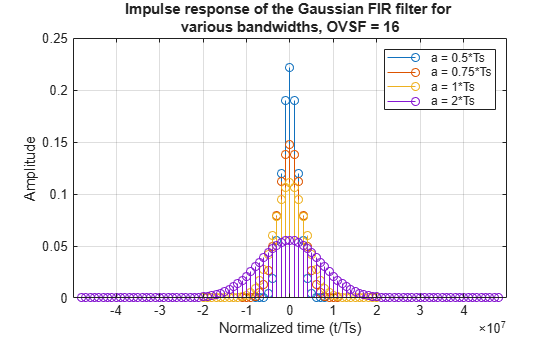### Frequency Response for FIR Gaussian Filter (oversampling factor=16)

We will calculate the frequency response for the Gaussian FIR filter with an oversampling factor of 16 and we will compare it with the ideal frequency response (i.e. frequency response of a continuous-time Gaussian filter).

```Fs = ovsf/Ts; fvtool(h(:,1),1,h(:,2),1,h(:,3),1,h(:,4),1,... 'FrequencyRange', 'Specify freq. vector', ... 'FrequencyVector',f,'Fs',Fs,'Color','white'); title('Ideal magnitude responses and FIR approximations, OVSF = 16') hold on plot(f*Ts,20*log10(Hideal),'--') hold off axis([0 32 -350 5]) legend(append(["B = " "Ideal, B = "],string(num2str(B','%g'))), ... 'NumColumns',2,'Location','best')```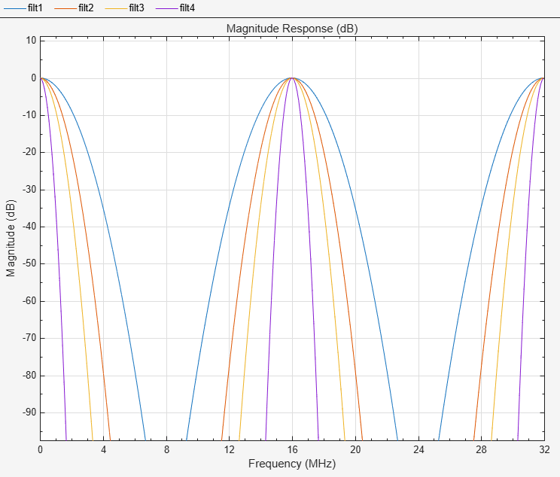Notice that the first two FIR filters exhibit aliasing errors and the last two FIR filters exhibit truncation errors. Aliasing occurs when the sampling frequency is not greater than the Nyquist frequency. In case of the first two filters, the bandwidth is large enough that the oversampling factor does not separate the spectral replicas enough to avoid aliasing. The amount of aliasing is not very significant however.

On the other hand, the last two FIR filters show the FIR approximation limitation before any aliasing can occur. The magnitude responses of these two filters reach a floor before they can overlap with the spectral replicas.

### Significance of the Oversampling Factor

The aliasing and truncation errors vary according to the oversampling factor. If the oversampling factor is reduced, these errors will be more severe, since this reduces the sampling frequency (thereby moving the replicas closer) and also reduces the filter lengths (increasing the error in the FIR approximation).

For example, if we select an oversampling factor of 4, we will see that all the FIR filters exhibit aliasing errors as the sampling frequency is not large enough to avoid the overlapping of the spectral replicas.

```ovsf = 4; % Oversampling factor (samples/symbol) h = zeros(25,4); iz = zeros(25,4); for k = 1:length(a) BT = B(k)*Ts; h(:,k) = gaussdesign(BT,span,ovsf); [iz(:,k),t] = impz(h(:,k)); end figure('Color','white') t = (t-t(end)/2)/Ts; stem(t,iz) title({'Impulse response of the Gaussian FIR filter'; 'for various bandwidths, OVSF = 4'}); xlabel('Normalized time (t/Ts)') ylabel('Amplitude') legend(sprintf('a = %g*Ts',a(1)/Ts),sprintf('a = %g*Ts',a(2)/Ts),... sprintf('a = %g*Ts',a(3)/Ts),sprintf('a = %g*Ts',a(4)/Ts)) grid on;```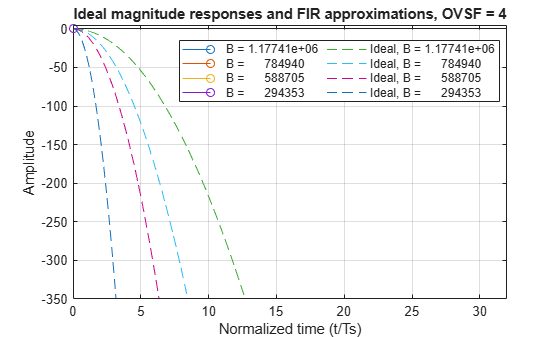### Frequency Response for FIR Gaussian Filter (oversampling factor=4)

We will plot and study the frequency response for the Gaussian FIR filter designed with oversampling factor of 4. A smaller oversampling factor means smaller sampling frequency. As a result, this sampling frequency is not enough to avoid the spectral overlap and all the FIR approximation filters exhibit aliasing.

```Fs = ovsf/Ts; fvtool(h(:,1),1,h(:,2),1,h(:,3),1,h(:,4),1,... 'FrequencyRange', 'Specify freq. vector', ... 'FrequencyVector',f,'Fs',Fs,'Color','white'); title('Ideal magnitude responses and FIR approximations, OVSF = 4') hold on plot(f*Ts,20*log10(Hideal),'--') hold off axis([0 32 -350 5]) legend(append(["B = " "Ideal, B = "],string(num2str(B','%g'))), ... 'NumColumns',2,'Location','southeast')```